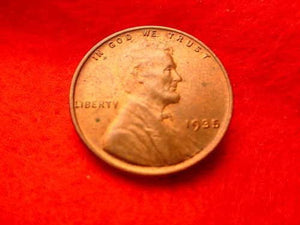# 1935 Lincoln Wheat Cent

Vendor
US Mint
Regular price
\$64.87
Sale price
\$62.70
Quantity must be 1 or more

• E

• a

• c

• h

• c

• o

• i

• n

• w

• i

• l

• l

• b

• e

• i

• n

• c

• i

• r

• c

• u

• l

• a

• t

• e

• d

• c

• o

• n

• d

• i

• t

• i

• o

• n

• .

• W

• i

• l

• l

• g

• r

• a

• d

• e

• G

• o

• o

• d

• t

• o

• V

• e

• r

• y

• G

• o

• o

• d

• o

• n

• t

• h

• e

• A

• N

• A

• G

• r

• a

• d

• i

• n

• g

• S

• c

• a

• l

• e

• .

• P

• l

• e

• a

• s

• e

• n

• o

• t

• e

• t

• h

• e

• c

• o

• i

• n

• p

• i

• c

• t

• u

• r

• e

• d

• i

• s

• n

• o

• t

• t

• h

• e

• s

• a

• m

• e

• c

• o

• i

• n

• y

• o

• u

• w

• i

• l

• l

• r

• e

• c

• e

• i

• v

• e

• d

• .

Each coin will be in circulated condition. Will grade Good to Very Good on the ANA Grading Scale. Please note the coin pictured is not the same coin you will received.